# Extremum Seeking Control for Reference Model Tracking of Uncertain Systems

This example shows the design of feedback and feedforward gains for a system, a common controller design technique. Here, you use an extremum seeking controller to track a given reference plant model by adapting feedback and feedforward gains for an uncertain dynamical system.

### Adaptive Gain Tuning for Uncertain Linear Systems

For this example, consider the following first-order linear system.

`$\underset{}{\overset{˙}{x}}\left(t\right)={a}_{0}x\left(t\right)+{b}_{0}u\left(t\right)$`

Here, $\mathit{x}\left(\mathit{t}\right)$ and $\mathit{u}\left(\mathit{t}\right)$ are the state and control input of the system, respectively. The constants ${\mathit{a}}_{0}$ and ${\mathit{b}}_{0}$ are unknown.

The goal of this example is to track the performance of the following reference plant model, which defines the required transient and steady-state behavior.

`${\underset{}{\overset{˙}{x}}}_{ref}\left(t\right)={a}^{*}{x}_{ref}\left(t\right)+{b}^{*}r\left(t\right)$`

Here, ${\mathit{x}}_{\mathrm{ref}}\left(\mathit{t}\right)$ is the state of the reference plant and $\mathit{r}\left(\mathit{t}\right)$ is the reference signal.

The aim of the control signal $u\left(t\right)$ is to make the states $x\left(t\right)$of the uncertain system track the reference states ${\mathit{x}}_{\mathrm{ref}}\left(\mathit{t}\right)$.

`$u\left(t\right)=Kx\left(t\right)-Kr\left(t\right)$`

The designed controller contains a feedback term, $\mathit{Kx}\left(\mathit{t}\right)$, and a feedforward term, $-\mathit{Kr}\left(\mathit{t}\right)$.

Substitute this control signal into the unknown linear system dynamics.

`$\underset{}{\overset{˙}{x}}\left(t\right)={a}_{0}x\left(t\right)+{b}_{0}\left(Kx\left(t\right)-Kr\left(t\right)\right)$`

You can rewrite this expression as shown in the following equation.

`$\underset{}{\overset{˙}{x}}\left(t\right)=\left({a}_{0}+{b}_{0}K\right)x\left(t\right)-{b}_{0}Kr\left(t\right)$`

In the ideal case, if the coefficients ${\mathit{a}}_{0}$ and ${\mathit{b}}_{0}$ of the nominal system dynamics are known, then you can determine the controller gain $\mathit{K}$ using pole-placement techniques. Doing so produces the following matching condition.

`${a}_{0}+{b}_{0}K={a}^{*},{b}_{0}K={b}^{*}$`

When you use a single gain value as both the feedforward and feedback gain, this matching condition might not be satisfied for all the possible values of ${\mathit{a}}_{0}$ and ${\mathit{b}}_{0}$. For a more general solution, you can tune two different gain values (multiparameter tuning).

For this example, use the following unknown system and reference dynamics.

`$\underset{}{\overset{˙}{x}}\left(t\right)=-1x\left(t\right)+u\left(t\right)$`

`${\underset{}{\overset{˙}{x}}}_{ref}\left(t\right)=-3{x}_{ref}\left(t\right)+2r\left(t\right)$`

In this case, the ideal control gain is $K=-2$.

### Extremum Seeking Control Adaptive Gain Tuning

To implement an extremum seeking control (ESC) approach to the preceding problem, you define an objective function, which the ESC controller then maximizes to find the controller gain $\mathit{K}$.

For this example, use the following objective function.

`$J=-10\int {\left(x\left(t\right)-{x}_{ref}\left(t\right)\right)}^{2}dt$`

The following figure shows the setup for extremum seeking control.

• The cost function is computed from the outputs of the reference system and the actual system.

• The extremum seeking controller updates the gain parameter.

• The control action is updated using the new gain value.

• This control action is applied to the actual system.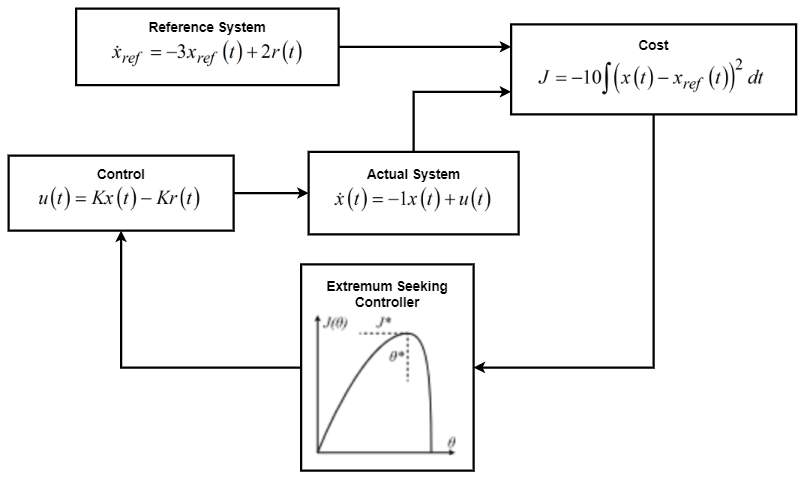The `firstOrderRefTracking_Esc` Simulink model implements this problem configuration.

```mdl = 'firstOrderRefTracking_Esc'; open_system(mdl)```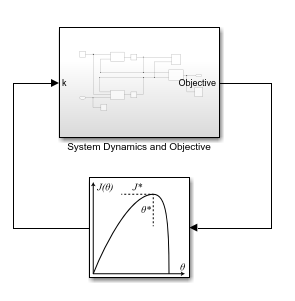In this model, you use the Extremum Seeking Control block to optimize the gain value.

The System Dynamics and Objective subsystem contains the reference model, the plant (including the actual system and control action), and the objective function computation. These elements are all implemented using MATLAB Function blocks.

`open_system([mdl '/System Dynamics and Objective'])`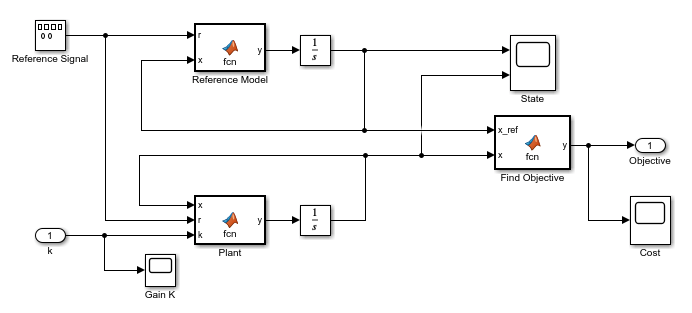Specify an initial guess for the gain value.

`IC = 0;`

The Extremum Seeking Control block perturbs the parameter value using a modulation signal. It then demodulates the resulting change in the objective function signal before computing the parameter update. Configure the extremum seeking control parameters for this block.

First specify the number of parameters to be tuned (`N`) and the learning rate (`lr`).

```N = 1; lr = 0.55;```

Configure the demodulation and modulation signals by specifying their frequency (`omega`), phases (`phi_1` and `phi_2`), and their amplitudes (`a` and `b`).

```omega = 5; % Forcing frequency a = 1; % Demodulation amplitude b = 0.1; % Modulation amplitude phi_1 = 0; % Demodulation phase phi_2 = 0; % Modulation phase```

For this example, the Extremum Seeking Control block is configured to remove high-frequency noise from the demodulated signal. Set the cutoff frequency for the corresponding low-pass filter.

`omega_lpf = 1;`

Simulate the model.

`sim(mdl);`

To check the reference tracking performance, view the state trajectories from the simulation. The actual trajectory converges to the reference trajectory in less than five seconds.

`open_system([mdl '/System Dynamics and Objective/State'])`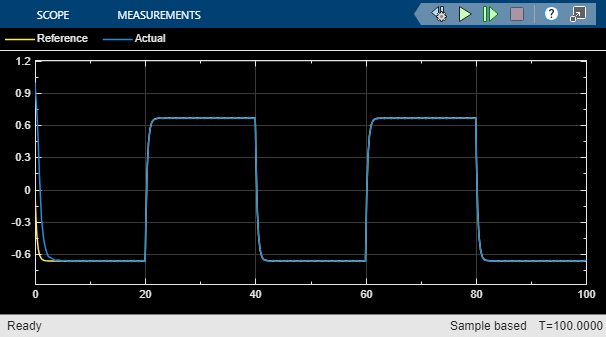To examine the behavior of the ESC controller, first view the objective function, which reaches its maximum value quickly.

`open_system([mdl '/System Dynamics and Objective/Cost'])`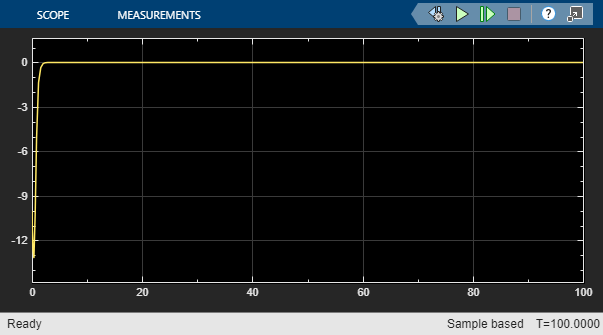By maximizing the objective function, the ESC controller optimizes the control gain value near its ideal value of –2. The fluctuations in the gain value are due to the modulation signal from the Extremum Seeking Control block.

`open_system([mdl '/System Dynamics and Objective/Gain K'])`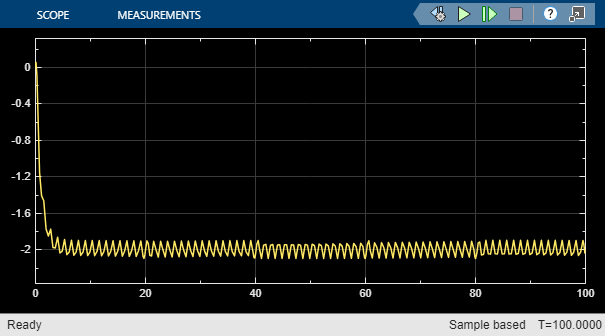`bdclose(mdl)`

## Related Topics